### Home > AC > Chapter 14 > Lesson 14.2.1.4 > Problem2-55

2-55.
1. For the function f(x) =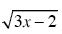find the value of each expression below. Homework Help ✎

1. f(1)

2. f(9)

3. f(4)

4. f(0)

5. What value of x makes f(x) = 6?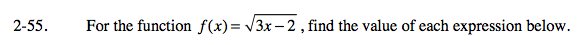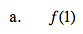Substitute 1 for x.

$f(1)=\sqrt{3-2}$

f(1) = 1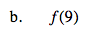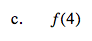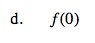The square root of a negative number is undefined.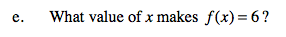Substitute 6 for f(x).

$6 = \sqrt{3\textit{x}-2}$

Square both sides.

36 = 3x − 2

Solve for x.

$\textit{x}=12\frac{2}{3}$